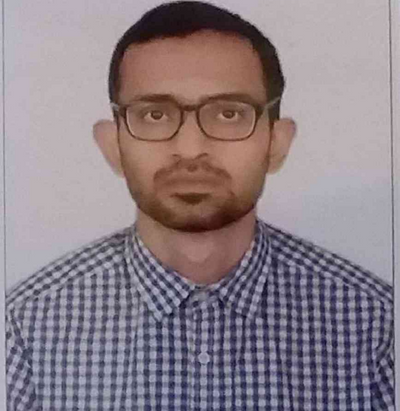## Debapratim Banerjee

Assistant Professor, Department of Mathematics

Ph.D. University of Pennsylvania

Dr Banerjee is a mathematician working on problems related to random matrix theory, mathematical physics, probability theory and mathematical statistics. Before coming to Ashoka university, he was an Inspire faculty fellow at the theoretical statistics and mathematics unit of the Indian Statistical Institute, Kolkata.

Academically, he did Bachelor’s (2010-2013) and Master’s (2013-2015) in statistics with a specialization in mathematical statistics and probability from Indian Statistical Institute, Kolkata and obtained his PhD degree from the department of statistics of the University of Pennsylvania, USA(2015-2019).

• Debapratim Banerjee. Asymptotic independence of largest eigenvalue and linear spectral statistics of Wigner matrices. Arxiv: https://arxiv.org/abs/2210.07878
• Debapratim Banerjee. A new combinatorial approach for Tracy-Widom law of Wigner matrices. Arxiv: https://arxiv.org/abs/2201.00300
• Debapratim Banerjee. Fluctuation of the free energy of Sherrington-Kirkpatrick model with Curie-Weiss interaction: the paramagnetic regime. Journal of Statistical Physics volume 178, pages 211–246 (2020).
• Debapratim Banerjee and Zongming Ma. Optimal signal detection in some spiked random matrix models: Likelihood ratio tests and linear spectral statistics. Ann. Statist. 50(4): 1910-1932.

#### Study at Ashoka

[current_url]